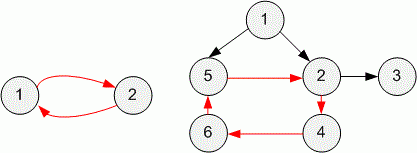favorite We need a little bit of your help to keep things running, click on this banner to learn more
Competitions

# Find a cycle

The directed and unweighted graph is given. Determine, does it contain a cycle. If yes, then print any of them.

#### Input

The first line contains two positive integers n and m (1n`105`, 1m`105`) - the number of vertices and edges in graph respectively. In the next m lines the edges are given. Each edge is described with a pair of numbers - the numbers of initial and final vertex respectively.

#### Output

If the graph does not contain the cycle, print "NO", otherwise print "YES" and then the list of vertices in the order of cycle traversal.Time limit 1 second
Memory limit 128 MiB
Input example #1
```2 2
1 2
2 1
```
Output example #1
```YES
1 2
```
Input example #2
```6 7
1 2
1 5
2 3
2 4
4 6
6 5
5 2
```
Output example #2
```YES
2 4 6 5
```
Source ЛКШ-2011 Севастополь 08.08.2011 д.1 1-я лига